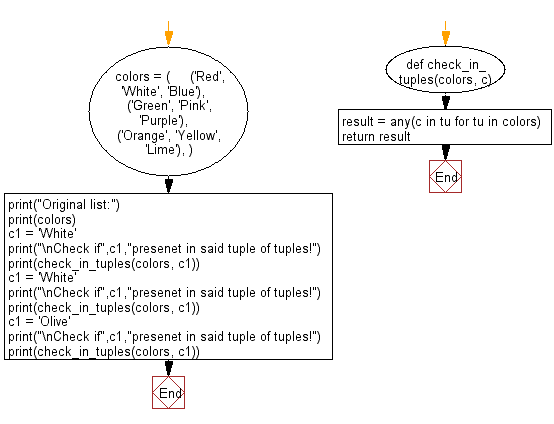﻿ Python: Check if a specified element presents in a tuple of tuples - w3resource# Python Exercise: Check if a specified element presents in a tuple of tuples

## Python tuple: Exercise-30 with Solution

Write a Python program to check if a specified element presents in a tuple of tuples.

Sample Solution:

Python Code:

``````def check_in_tuples(colors, c):
result = any(c in tu for tu in colors)
return result

colors = (
('Red', 'White', 'Blue'),
('Green', 'Pink', 'Purple'),
('Orange', 'Yellow', 'Lime'),
)
print("Original list:")
print(colors)
c1 = 'White'
print("\nCheck if",c1,"presenet in said tuple of tuples!")
print(check_in_tuples(colors, c1))
c1 = 'White'
print("\nCheck if",c1,"presenet in said tuple of tuples!")
print(check_in_tuples(colors, c1))
c1 = 'Olive'
print("\nCheck if",c1,"presenet in said tuple of tuples!")
print(check_in_tuples(colors, c1))
```
```

Sample Output:

```Original list:
(('Red', 'White', 'Blue'), ('Green', 'Pink', 'Purple'), ('Orange', 'Yellow', 'Lime'))

Check if White presenet in said tuple of tuples!
True

Check if White presenet in said tuple of tuples!
True

Check if Olive presenet in said tuple of tuples!
False
```

Flowchart:## Visualize Python code execution:

The following tool visualize what the computer is doing step-by-step as it executes the said program:

Python Code Editor:

Have another way to solve this solution? Contribute your code (and comments) through Disqus.

What is the difficulty level of this exercise?

Test your Programming skills with w3resource's quiz.

﻿

## Python: Tips of the Day

Decapitalizes the first letter of a string:

Example:

```def tips_decapitalize(s, upper_rest=False):
return s[:1].lower() + (s[1:].upper() if upper_rest else s[1:])
print(tips_decapitalize('PythonTips'))
print(tips_decapitalize('PythonTips', True))
```

Output:

```pythonTips
pYTHONTIPS
```